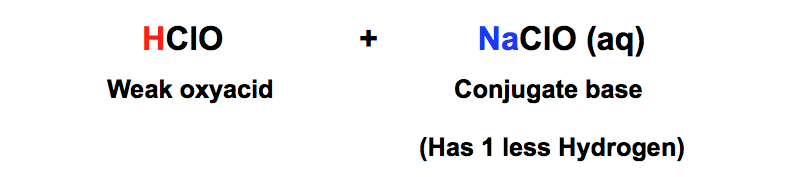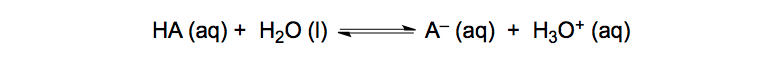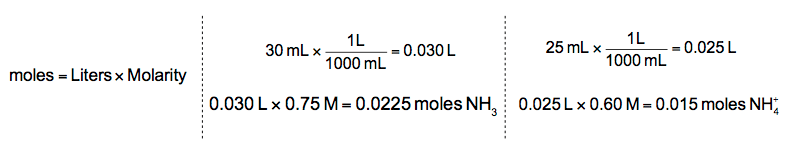Ch.16 - Aqueous Equilibrium WorksheetSee all chapters
 Ch.1 - Intro to General Chemistry 2hrs & 53mins 0% complete Worksheet Ch.2 - Atoms & Elements 2hrs & 49mins 0% complete Worksheet Ch.3 - Chemical Reactions 3hrs & 25mins 0% complete Worksheet BONUS: Lab Techniques and Procedures 1hr & 38mins 0% complete Worksheet BONUS: Mathematical Operations and Functions 47mins 0% complete Worksheet Ch.4 - Chemical Quantities & Aqueous Reactions 3hrs & 30mins 0% complete Worksheet Ch.5 - Gases 3hrs & 47mins 0% complete Worksheet Ch.6 - Thermochemistry 2hrs & 28mins 0% complete Worksheet Ch.7 - Quantum Mechanics 2hrs & 35mins 0% complete Worksheet Ch.8 - Periodic Properties of the Elements 1hr & 57mins 0% complete Worksheet Ch.9 - Bonding & Molecular Structure 2hrs & 5mins 0% complete Worksheet Ch.10 - Molecular Shapes & Valence Bond Theory 1hr & 31mins 0% complete Worksheet Ch.11 - Liquids, Solids & Intermolecular Forces 3hrs & 40mins 0% complete Worksheet Ch.12 - Solutions 2hrs & 17mins 0% complete Worksheet Ch.13 - Chemical Kinetics 2hrs & 22mins 0% complete Worksheet Ch.14 - Chemical Equilibrium 2hrs & 26mins 0% complete Worksheet Ch.15 - Acid and Base Equilibrium 4hrs & 42mins 0% complete Worksheet Ch.16 - Aqueous Equilibrium 3hrs & 48mins 0% complete Worksheet Ch. 17 - Chemical Thermodynamics 1hr & 44mins 0% complete Worksheet Ch.18 - Electrochemistry 2hrs & 58mins 0% complete Worksheet Ch.19 - Nuclear Chemistry 1hr & 33mins 0% complete Worksheet Ch.20 - Organic Chemistry 3hrs 0% complete Worksheet Ch.22 - Chemistry of the Nonmetals 2hrs & 1min 0% complete Worksheet Ch.23 - Transition Metals and Coordination Compounds 1hr & 54mins 0% complete Worksheet

# Henderson Hasselbalch Equation

See all sectionsJules Bruno

The Henderson Hasselbalch Equation is the equation that can be used to determine the pH of a buffer solution by relating pKa and the molar concentrations of a weak acid and its conjugate base.

What is a Buffer?

A buffer is a solution comprised of a weak acid (HA) and its conjugate base (A). An example of a buffer is hypochlorous acid, HClO, and its conjugate base of sodium hypochlorite, NaClO.HA/A- Buffer Solution

A buffer can resist significant changes in pH by keeping H+ and OH ions constant. How is this possible?

When a strong acid is added the conjugate base of the buffer will neutralize it.NaClO, HCl Strong Acid-Weak Base Neutralization Equation

When a strong base is added the weak acid of the buffer will neutralize it.HClO, NaOH Strong Base-Weak Acid Neutralization Equation

As long as the weak acid and conjugate base components of the buffer are greater in amount than the strong acid or strong base then the buffer will be maintained.

Deriving the Henderson Hasselbalch Equation

When a weak acid dissociates in water an equilibrium is established:Chemical Equilibrium Equation (Weak Acid)

From this equation we can set up our equilibrium expression with the use of our acid dissociation constant, Ka. Remember that we ignore solids and liquids.Ka Equilibrium Expression (Weak Acid)

By rearranging our equilibrium expression we obtain the following format:Derived Ka Equilibrium Expression (Weak Acid)

By taking the –log of both sides we obtain:Deriving the Henderson Hasselbalch Buffer Equation

By inverting the ratio HA and A we finally obtain the Henderson Hasselbalch Equation:Henderson Hasselbalch Buffer Equation

The values of HA and A within the Henderson Hasselbalch Equation can either be in moles or molarity.

Using the Henderson Hasselbalch Equation

The Henderson Hasselbalch Equation is a convenient way to calculate the pH of a buffer solution.

PRACTICE 1: Calculate the pH of a solution that is 0.27 M in HF and 0.11 M in NaF. The acid dissociation constant of HF is 3.5 x 10-4

STEP 1: Identify the weak acid and its conjugate base.

Since the Ka value of HF is less than 1 it represents the weak acid and because NaF possesses 1 less hydrogen than HF it represents the conjugate base.

STEP 2: Take the –log of Ka to determine the pKa value.Determining and calculating pKa

STEP 3: Plug in your given and calculated values into the Henderson Hasselbalch Equation.HF, NaF Henderson Hasselbalch Buffer Equation, pH, pKa and molarity

Now what happens when we are given the volume and molarity of the buffer components?

PRACTICE 2: Calculate the pH of a buffer solution that is 25.0 mL of 0.60 M in NH4+ and 30.0 mL of 0.75 M in NH3. (Kb = 1.8 × 10−5 for NH3)

STEP 1: Identify the weak acid and its conjugate base.

Another way of looking at buffer is it being composed of a conjugate acid and its weak base. Since the Kb value of NH3 is less than 1 it represents the weak base and because NH4+ possesses 1 more hydrogen than NH3 it represents the conjugate acid.

STEP 2: Change Kb into KaKb to Ka Conversion

STEP 3: Take the –log of Ka to determine the pKa value.Determining pKa from Ka

STEP 4: When given both the volume and molarity of the buffer components we must calculate their moles.Determining moles from L and M

STEP 5: Plug in your given and calculated values into the Henderson Hasselbalch Equation.NH4+, NH3 Henderson Hasselbalch Buffer Equation and moles

The Henderson Hasselbalch Equation is a useful tool in determining the pH of a buffer solution without the use of an ICE Chart. This equation will also play a role in acid-base identificationacid-base titrations and titration curves.Jules Bruno

Jules felt a void in his life after his English degree from Duke, so he started tutoring in 2007 and got a B.S. in Chemistry from FIU. He’s exceptionally skilled at making concepts dead simple and helping students in covalent bonds of knowledge.